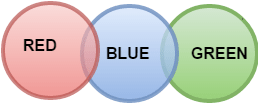# Logical Reasoning for Bank and other Government exams - Set 1

In a certain code language, ‘sea is deep’ means ‘213’, ‘sky is blue’ means ‘514’ and ‘sea looks blue’ means ‘264’

What number is the code for ‘blue’?

a. 1
b. 2
c. 3
d. 4
e. 5
 Answer  Explanation ANSWER: 4 Explanation: In the first and second statements, the common digit is ‘1’ and the common word is ‘is’.So, ‘1’ is the code for ‘is’.In the second and third statements, the common digit is ‘4’ and the common word is ‘blue’.So, ‘4’ is the code for ‘blue’.Thus, in the second statement, ‘5’ is the code for ‘sky’.In the first and third statements, the common digit is ‘2’ and the common word is ‘sea’.So, ‘2’ is the code for ‘sea’.Thus, in the first statement, ‘3’ is the code for ‘deep’.In the third statement, ‘6’ is the code for ‘looks’.

In a certain code language, ‘sea is deep’ means ‘213’, ‘sky is blue’ means ‘514’ and ‘sea looks blue’ means ‘264’

What number is the code for ‘deep’?

a. 1
b. 2
c. 3
d. 4
e. 5
 Answer  Explanation ANSWER: 3 Explanation: In the first and second statements, the common digit is ‘1’ and the common word is ‘is’.So, ‘1’ is the code for ‘is’.In the second and third statements, the common digit is ‘4’ and the common word is ‘blue’.So, ‘4’ is the code for ‘blue’.Thus, in the second statement, ‘5’ is the code for ‘sky’.In the first and third statements, the common digit is ‘2’ and the common word is ‘sea’.So, ‘2’ is the code for ‘sea’.Thus, in the first statement, ‘3’ is the code for ‘deep’.In the third statement, ‘6’ is the code for ‘looks’.

3)   There are 64 players in a knock out tournament and every player is ranked (seeded) from 1 - 64.

The matches are played in such a manner that in round one the 1st seeded player plays with the 64th, 2nd with the 63rd and so on.
The players who win move on to the next round whereas others are out of the competition.

In second round, the winner of match 1 will play winner of the last match (which was between seed 32 and seed 33), and winner of match 2 will meet the winner of second last match in round 1 and so forth.

Thus, after all rounds winner is declared.

Which seeds will play Match no 7 and Match no 10 in Round 1 of a 32-player tournament?

a. 26, 23
b. 27, 24
c. 28, 25
d. 29, 26
 Answer  Explanation ANSWER: 26, 23 Explanation: For solving these type of problems - Notice that the sum of the seeding in every match will be equal to total players + 1. i.e. 1 + 32 = 33, 2 + 31 = 33.In round of 64, sum of seeds will be 65, and in round of 16, sum of seeds will be 17. And so forth.Thus, for 32 player tournaments, it will be 32 + 1 = 33.For, match 7, opponent will be 33 -7 = 26Similarly, for match 10, opponent will be 23.

4)   Choose the odd pair of words

a. Bass - Shoal
b. Albatross – Rookery
c. Coyotes – Band
d. Man – Woman
e. Bee – Swarm
 Answer  Explanation ANSWER: Man – Woman Explanation: In all other pairs, second term is the collective group of the first.

5)   Choose the odd pair.

b. Large – Big
c. Heavy - Light
d. Small - Tiny
 Answer  Explanation ANSWER: Heavy - Light Explanation: All other pairs are synonyms of each other.

1. Rage, Raider, Rattler, Razor, Reaper, Rictor and Rogue are sitting in a circle facing at the centre.
2. Reaper is the neighbour Rage and Razor.
3. Rogue is not between Rictor and Rattler.
4. Rictor is to the immediate right of Rage.

Which of the following pairs are not adjacent to each other?

a. Raider Rage
b. Rattler Raider
c. Razor Reaper
d. Rogue Razor
 Answer  Explanation ANSWER: Raider Rage Explanation: In “Circular Seating Arrangement” when all the persons are facing inwards or center - thenPersons to his/her (specific person) left are in anti-clockwise direction.Persons to his/her (specific person) right are in clockwise directionRictor is to immediate right of Rage.Reaper is the neighbor of Rage and Razor i.e. Reaper is between Rage and RazorRogue is not between Rictor and Rattler. So, Raider is between Rictor and Rattler.Thus, the arrangement is as shown -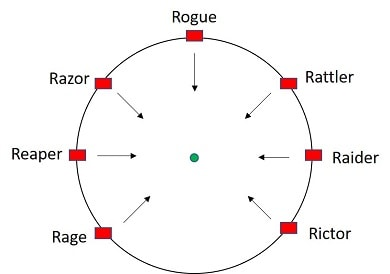Each one of Rattler-Raider, Razor-Reaper, and Rogue-Razor is a pair of persons sitting adjacent to each other, while Raider-Rage is not.

1. Rage, Raider, Rattler, Razor, Reaper, Rictor and Rogue are sitting in a circle facing at the centre.
2. Reaper is the neighbour Rage and Razor.
3. Rogue is not between Rictor and Rattler.
4. Rictor is to the immediate right of Rage.

Which of the following pairs has the second person sitting immediately to two places the right of the first?

a. Rage Raider
b. Rattler Raider
c. Reaper Rage
d. Razor Rogue
 Answer  Explanation ANSWER: Rage Raider Explanation: Rictor is to immediate right of Rage.Reaper is the neighbor of Rage and Razor i.e. Reaper is between Rage and RazorRogue is not between Rictor and Rattler. So, Raider is between Rictor and Rattler.Thus, the arrangement is as shown -Raider is second to the right of Rage, while Rogue is to the immediate left of Razor.Rage sits immediately to the right of Reaper.

8)   In each of the following questions, one term in the number series is wrong. Find the wrong term.

4, 7, 9, 10, 13, 15, 16, 20

a. 7
b. 15
c. 16
d. 20
 Answer  Explanation ANSWER: 20 Explanation: The pattern in the given series is +3 +2 +1 +3 +2 + 1 …….The wrong term is 20The correct term should be 16 + 3 = 19

9)   In each of the following questions, one term in the number series is wrong. Find the wrong term.

1, 5, 11, 19, 29, 41, 56

a. 54
b. 55
c. 56
d. 57
 Answer  Explanation ANSWER: 56 Explanation: The correct sequence here is +4 +6 +8 +10 + 12 ….The wrong term is 56The next term should be 41 + 14 = 55

10)   In the question below is given a statement followed by two courses of action numbered I and II. You have to assume everything in the statement to be true and based on the information given in the statement, decide which of the suggested courses of action logically follow(s) for pursuing.

(A) If only I follows
(B) If only II follows
(C) If either I or II follows
(D) If neither I nor II follow
(E) If both I and II follow.

Statement - People residing in some rural areas do not get fair opportunities of education.

Courses of Action - I. Government should open more schools in rural areas.

II. Awareness programme must be conducted in these areas.

a. Only I follow
b. Only II follow
c. Either I or II follow
d. Neither I nor II follows
e. Both follow
 Answer  Explanation ANSWER: Both follow Explanation: People in rural areas should be made aware of the need of education and persuaded to send children to schools. Also, proper facilities to render education must also be available in the form of schools and colleges.So, both courses follow here.

11)   In the question below is given a statement followed by two courses of action numbered I and II. You have to assume everything in the statement to be true and based on the information given in the statement, decide which of the suggested courses of action logically follow(s) for pursuing.

(A) If only I follows
(B) If only II follows
(C) If either I or II follows
(D) If neither I nor II follow
(E) If both I and II follow.

Statement - Rehabilitation process of over 12000 freed slaves has slowed down extremely.

Courses of Action - I. The slaves should not be freed.

II. The impediments in the way of proper rehabilitation of these people should be removed.

a. Only I follows
b. Only II follows
c. Either I or II follows
d. Neither I nor II follows
e. Both I and II follow
 Answer  Explanation ANSWER: Only II follows Explanation: The obstacles in the way of rehabilitation must be removed in a speedy manner. So, only course II follows here.

12)   In the following question, a statement/group of statements is given followed by some conclusions. Without resolving anything yourself, choose the conclusion which logically follows from the statement(s).

Statement - Ability is poor man’s wealth.

a. A rich man is always able.
b. A rich man does not have the ability to earn money.
c. A poor man is not always able.
d. A poor man can earn wealth if he has ability.
 Answer  Explanation ANSWER: A poor man can earn wealth if he has ability. Explanation: No explanation is available for this question!

13)   In the following question, a statement/group of statements is given followed by some conclusions. Without resolving anything yourself, choose the conclusion which logically follows from the statement(s).

Statement - Good Samaritans always help the accident victims.

a. People who help the victims are bad samaritans.
b. Doctors are Good Samaritans.
c. Some men who are kind-hearted do not help the victims.
d. Those who help people in need are Good Samaritans.
 Answer  Explanation ANSWER: Those who help people in need are Good Samaritans. Explanation: No explanation is available for this question!

• Popeye - the Sailor man started from his boat towards west.
• After covering a distance of 250 m, he turned to the right and walked 100 m.
• He then again turned to the right and walked 150 m.
• Then, he turned right at 135° and covered 300 m.
Which direction is he facing now?

a. South-East
b. South-West
c. North-East
d. North-West
 Answer  Explanation ANSWER: South-West Explanation: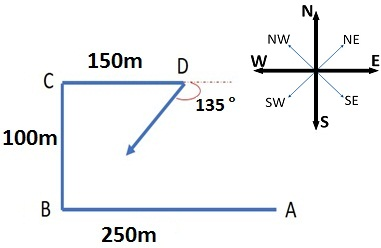• Sandeep Nath runs 40 m towards East and turns to right and runs 20 m.
• Then he turns to the right and runs 18 m.
• Again, he turns to right and runs 10 m.
• After this, he turns to left and runs 24 m and finally he turns to right and 12 m.
Which direction is Sandeep Nath facing?

a. East
b. West
c. South
d. North
 Answer  Explanation ANSWER: North Explanation: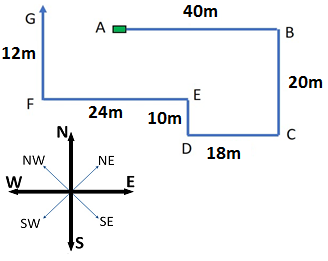1. In a family of six persons, L, M, N, F, P, Q, there are two married couples.
2. F is grandmother of L and mother of M.
3. N is wife of M and mother of Q.
4. Q is the grand-daughter of P.

How many male members are there in the family?

a. 1
b. 2
c. 3
d. Cannot be determined
 Answer  Explanation ANSWER: Cannot be determined Explanation: Here, the gender of L cannot be determined.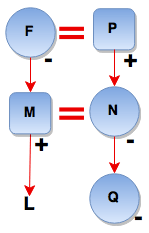1. In a family of six persons, L, M, N, F, P, Q, there are two married couples.
2. F is grandmother of L and mother of M.
3. N is wife of M and mother of Q.
4. Q is the grand-daughter of P.

Which of the following is true?

a. L is the brother of Q.
b. L is the sister of Q.
c. M has three daughters.
d. None of these
 Answer  Explanation ANSWER: None of these Explanation: Here, the gender of L cannot be determined. So, nothing can be predicted about it.18)   When the time in the clock is 7.20 pm, then the angle between the hands of the clock is

a. 80
b. 90
c. 100
d. 110
 Answer  Explanation ANSWER: 100 Explanation: At 7 o’ clock, the hour hand is at 210 degrees from the vertical.In 20 minutes,Hour hand = 210 + 20 x (0.5) = 220 (The hour hand moves at 0.5 dpm)Minute hand = 20 x (6) = 120 (The minute hand moves at 6 dpm)Difference or angle between the hands = 220 - 120 = 100 degree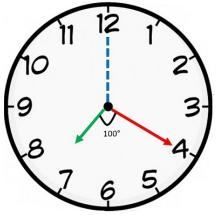19)   In the question below is given a statement followed by two conclusions numbered I and II.

(A) If only I follows
(B) If only II follows
(C) If either I or II follows
(D) If neither I nor II follows
(E) If both I and II follow.

Statement - I. Some dolls can speak.
Conclusion - I. Bad toys can speak.

a. Only I follows
b. Only II follows
c. Either I or II follows
d. Neither I nor II follows
e. Both I and II follow
 Answer  Explanation ANSWER: Neither I nor II follows Explanation: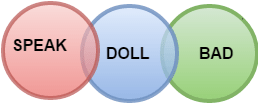20)   In the following question consists of figures marked A, B, C, D called the Problem Figures followed by other figures marked 1, 2, 3, 4 called the Answer Figures.

Select a figure from amongst the Answer Figures which will continue the same series as established by the Problem Figures.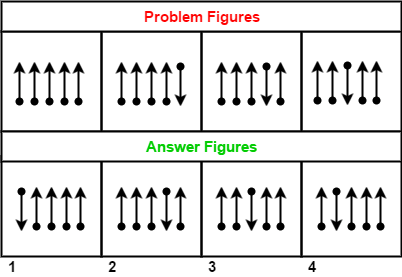a. 1
b. 2
c. 3
d. 4
 Answer  Explanation ANSWER: 4 Explanation: The movement of arrows is from right to left in a set order. In the next step, it is inverted vertically and moves one step forward as shown.The answer figure should be figure 4.

21)   Each of the following questions consists of figures marked A, B, C, D called the Problem Figures followed by other figures marked 1, 2, 3, 4 called the Answer Figures.

Select a figure from amongst the Answer Figures which will continue the same series as established by the Problem Figures.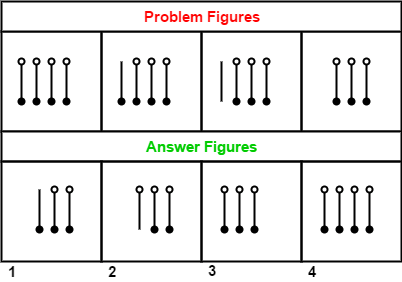a. 1
b. 2
c. 3
d. 4
 Answer  Explanation ANSWER: 1 Explanation: Elements are deleted one by one beginning from top-left bar.Each line has two elements (circle) attached to it and each element is deleted one by one from left to right. The answer figure should be figure 1.

22)   In the question below is given a statement followed by two conclusions numbered I and II.

(A) If only I follows
(B) If only II follows
(C) If either I or II follows
(D) If neither I nor II follows
(E) If both I and II follow.

Statement - I. Some lions are bats.
II. Some bats are cat.

Conclusion - I. All lions are cat.
II. No cat are bats.

a. Only I follows
b. Only II follows
c. Either I or II follows
d. Neither I nor II follows
e. Both I and II follow
 Answer  Explanation ANSWER: Neither I nor II follows Explanation: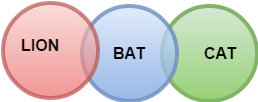23)   In the question below is given a statement followed by two conclusions numbered I and II.

(A) If only I follows
(B) If only II follows
(C) If either I or II follows
(D) If neither I nor II follows
(E) If both I and II follow.

Statement - I. Some red are blue.
II. Some blue are green.

Conclusion - I. No green are blue.
II. Some green are blue.

a. Only I follows
b. Only II follows
c. Either I or II follows
d. Neither I nor II follows
e. Both I and II follow
 Answer  Explanation ANSWER: Only II follows Explanation: